# Function Handles and Anonymous Functions

A function handle is a MATLAB data type that represents a function. A typical use of function handles is to pass a function to another function. For example, you can use function handles as input arguments to functions that evaluate mathematical expressions over a range of values.
A function handle is specified with an '@' sign:
For example to compute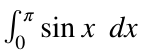, run
f=@sin;
I = integral(f,0,pi)
I = 2.0000
We can also make a function handle for a function cubic we created (see the bottom of the page):
I2 = integral(@cubic,0,1)
I2 = 0.2500

#### Anonymous Functions

We can define a function without a function statement using anonymous functions, which are defined using the following syntax,
I3 = 0.1667
Note that we can also define anonymous functions with two inputs, but any anonymous function defined on the command line can only have one output
f=@(x,y)(x^2+y^2);
Now, why would we want to use an anonymous function? It's a way to pass a function with parameters. The function sina.m defined below is a function of two variables but the builtin matlab function integral expects to receive a function of one variable. By using the anonymous function defined inside the loop below, we can repeatedly define functions of one variable x dependent on the parameter a=k/n.
n=10;
y=zeros(1,n);
for k=1:n
f=@(x)sina(x,k/n);
y(k)=integral(f,0,pi);
end
plot(1:n,y)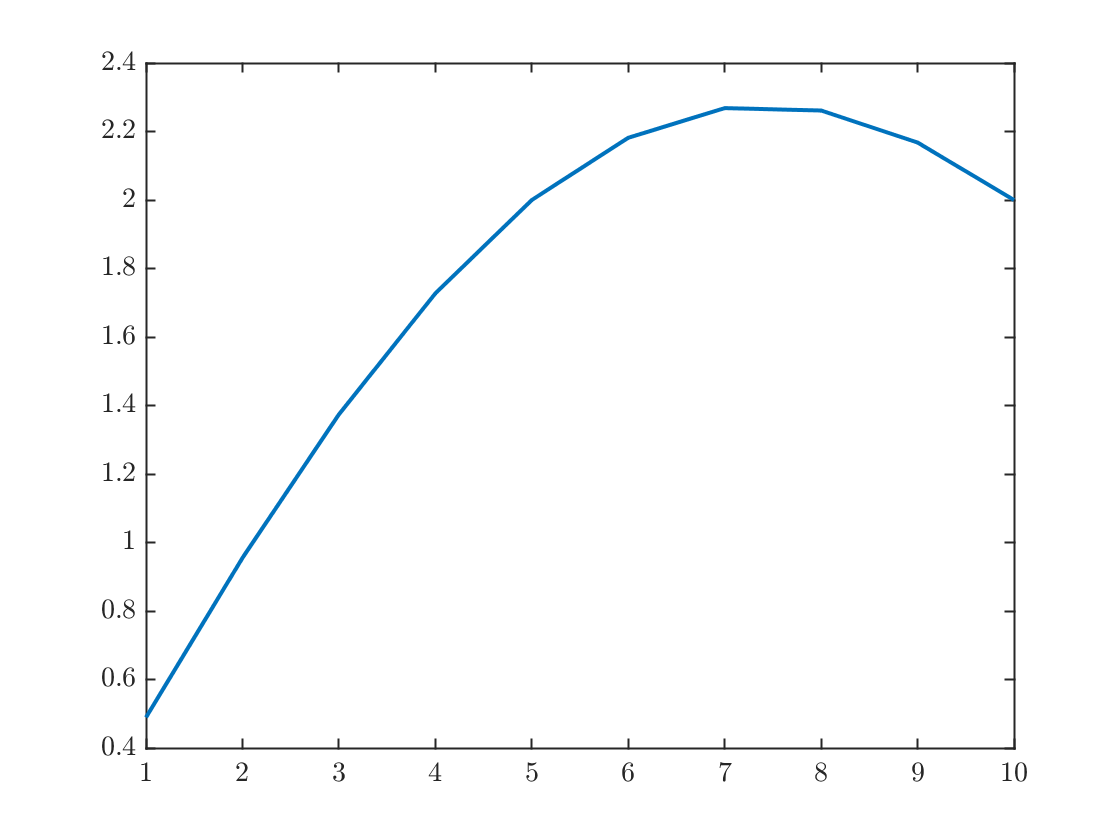Lots more information on function handles and anonymous functions is available here.
Pro tip: Look at the loop above. Note that my first step was to declare a variable n=10. This variable gets called several more times. That way, if I decide to change n later, I don't have to sort through my code and find all the places where I used the "magic number" 10. Most likely I would miss one of them, and this would lead to an error. Programmers call this "avoiding magic numbers" and it's important for readability and for avoiding errors.

## Inverting Functions:

We can use function handles to solve equations. For example, suppose we wanted to invert the sine function. Of course MATLAB has inverse trig functions built in, but pretend it didn't. We can define an anonymous matlab function, plot it and find its zero.
sinb=@(x,b)(sin(x)-b);
x=linspace(-pi,pi);
ff=@(x)sinb(x,1/2);
plot(x,ff(x));xlabel('$x$');ylabel('$\sin{x}-\frac{1}{2}$')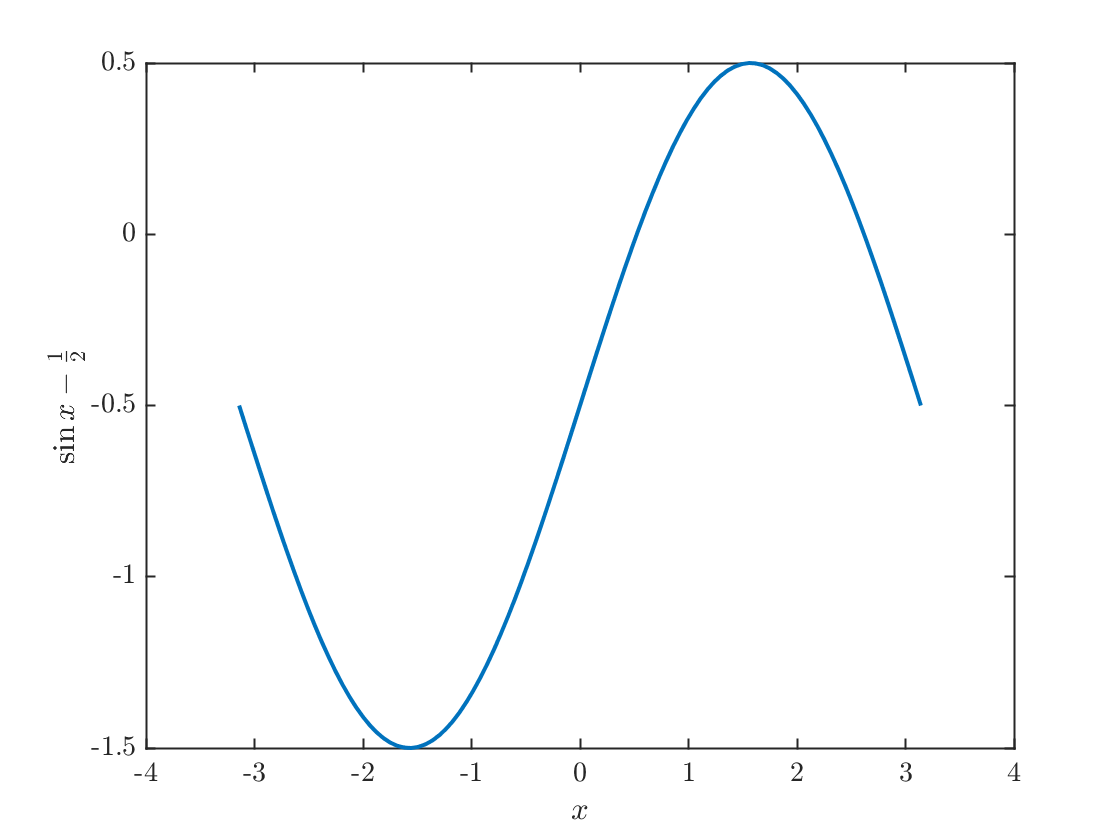This has two roots, one near x=0.5 and one near x=2.5. Let's try to find these using the fzero function:
x1=fzero(ff,0.5); % Try to solve ff=0 near 0.5
x2=fzero(ff,2.5); % Try to solve ff=0 near 2.5
plot(x,ff(x),x1,ff(x1),'x',x2,ff(x2),'o')
xlabel('$x$');ylabel('$\sin{x}-\frac{1}{2}$')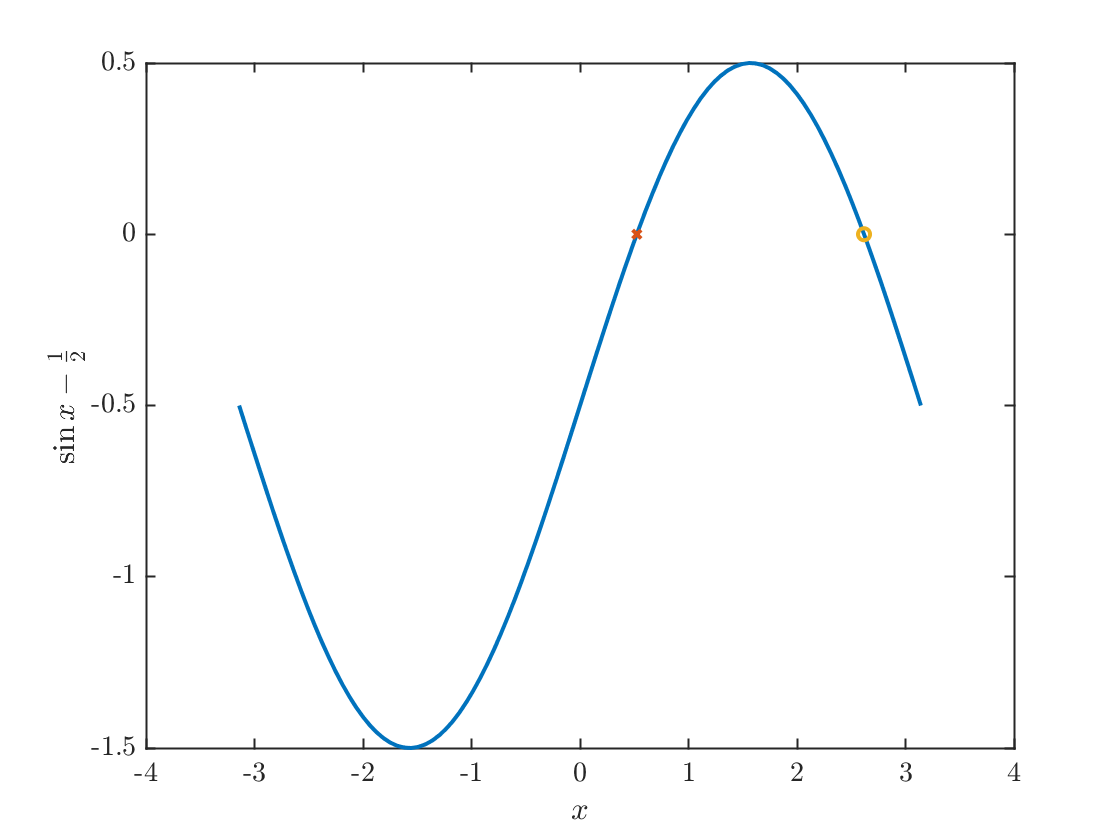## For more details

See this page, especially the three Topics entitled "Create Function Handles", "Pass Function to Another Function", and "Parameterizing Functions"

The Lambert W function is defined as the inverse of the function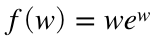,
x1=linspace(-3,-1);x2=linspace(-1,1.5);
plot(x1,x1.*exp(x1),'--',x2,x2.*exp(x2));
xlabel('$w$');ylabel('$f(w)$')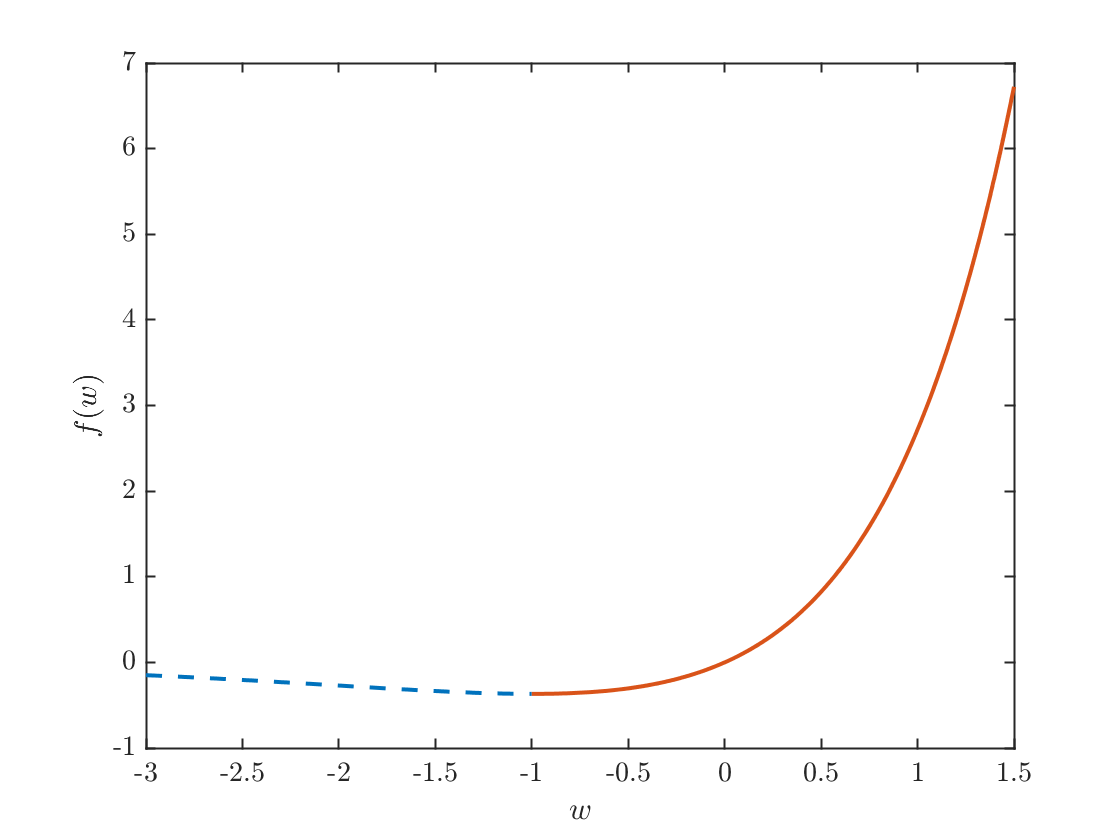Note that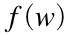has an absolute minimum at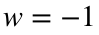andso the inverse is defined for(The solid part of the curve in the figure.)
Problem 1: Write a program to calculate the Lambert function which is denoted as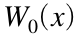and use it to calculate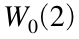. Hint: you can use 0 as the initial guess for the rootfinder.
Problem 2: Find all the roots of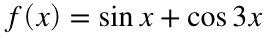on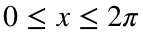.

## External functions

Usually, these would be contained in separate files with filenames matching the function names. That is cubic.m and sina.m, but for demonstration purposes, it is easier to place them in the same file.
A cubic function:
function y = cubic(x)
y=x-x.^3;
end
A function of two variables. If we want to study bifurcations, we need these!
function y = sina(x,a)
y=sin(a*x);
end